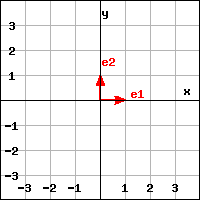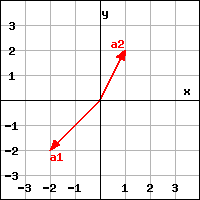Suppose $f(\mathbf{e_n}) = \mathbf{a_n}$ for $n = 1, 2$ and $f$ is a linear transformation.$\Large \stackrel{f}{\to}$Domain Codomain
1. Find a formula for $f$. Your answer should be a coordinate vector with the variables $x$ and $y$ in its components.
$f(x,y) =$
2. Find the matrix for the linear transformation $f$ (relative to the standard basis in the domain and codomain). That is, find the matrix $A$ such that $f(\mathbf{x}) = A \mathbf{x}$. For instance, enter [ [1,2], [3,4] ] for the matrix ${\left[\begin{array}{cc} 1 &2\cr 3 &4 \end{array}\right]}$.
$A=$
3. Find the kernel of $f$. Enter your answer as a vector with constant entries, a vector with the variables $x$ or $y$ (or both) in its components (using a minimum number of variables), or enter R^2 for $\mathbb{R}^2$.
$\mathrm{ker}(f) =$
4. Find the image of $f$. Enter your answer as a vector with constant entries, a vector with the variables $x$ or $y$ (or both) in its components (using a minimum number of variables), or enter R^2 for $\mathbb{R}^2$.
$\mathrm{im}(f) =$
5. The linear transformation $f$ is (select all that apply):

You can earn partial credit on this problem.